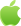# python 函数内部修改外部变量的方法```

x = 0

global x

x += 1

print(x) # 输出 1

```

```

def make_counter():

count = 0

def counter():

nonlocal count

count += 1

return count

return counter

counter1 = make_counter()

counter2 = make_counter()

print(counter1()) # 输出 1

print(counter1()) # 输出 2

print(counter2()) # 输出 1

print(counter2()) # 输出 2

```

```

my_list = []

my_list.append(item)

print(my_list) # 输出 [1, 2, 3]

```

```

x = 0

x = 1 # 这里定义了一个和全局变量 x 相同的变量

x += 1

print(x) # 输出 0

```

【原创声明】凡注明“来源：优草派”的文章，系本站原创，任何单位或个人未经本站书面授权不得转载、链接、转贴或以其他方式复制发表。否则，本站将依法追究其法律责任。Python兼职赚钱

2022-03-29 更新Android下载